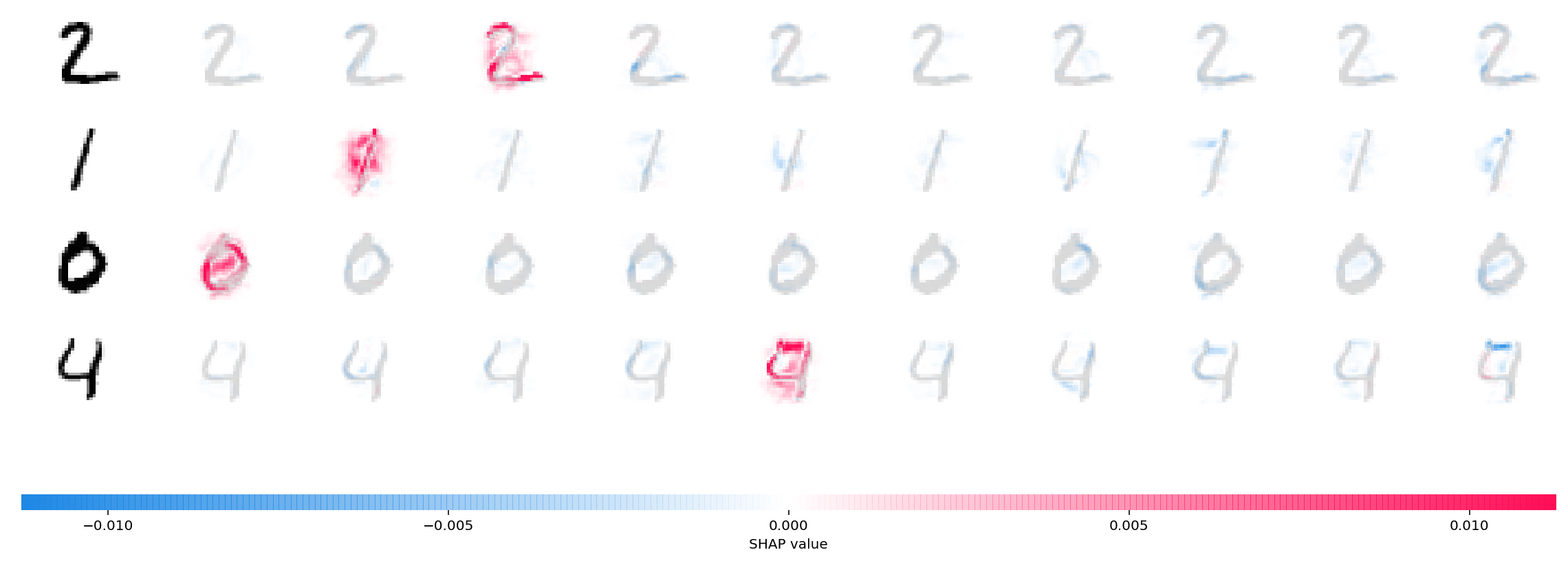# Front Page DeepExplainer MNIST Example¶

A simple example showing how to explain an MNIST CNN trained using Keras with DeepExplainer.

In :
# this is the code from https://github.com/keras-team/keras/blob/master/examples/mnist_cnn.py
from __future__ import print_function
import keras
from keras.datasets import mnist
from keras.models import Sequential
from keras.layers import Dense, Dropout, Flatten
from keras.layers import Conv2D, MaxPooling2D
from keras import backend as K

batch_size = 128
num_classes = 10
epochs = 12

# input image dimensions
img_rows, img_cols = 28, 28

# the data, split between train and test sets
(x_train, y_train), (x_test, y_test) = mnist.load_data()

if K.image_data_format() == 'channels_first':
x_train = x_train.reshape(x_train.shape, 1, img_rows, img_cols)
x_test = x_test.reshape(x_test.shape, 1, img_rows, img_cols)
input_shape = (1, img_rows, img_cols)
else:
x_train = x_train.reshape(x_train.shape, img_rows, img_cols, 1)
x_test = x_test.reshape(x_test.shape, img_rows, img_cols, 1)
input_shape = (img_rows, img_cols, 1)

x_train = x_train.astype('float32')
x_test = x_test.astype('float32')
x_train /= 255
x_test /= 255
print('x_train shape:', x_train.shape)
print(x_train.shape, 'train samples')
print(x_test.shape, 'test samples')

# convert class vectors to binary class matrices
y_train = keras.utils.to_categorical(y_train, num_classes)
y_test = keras.utils.to_categorical(y_test, num_classes)

model = Sequential()
model.add(Conv2D(32, kernel_size=(3, 3),
activation='relu',
input_shape=input_shape))
model.add(Conv2D(64, (3, 3), activation='relu'))
model.add(MaxPooling2D(pool_size=(2, 2)))
model.add(Dropout(0.25))
model.add(Flatten())
model.add(Dense(128, activation='relu'))
model.add(Dropout(0.5))
model.add(Dense(num_classes, activation='softmax'))

model.compile(loss=keras.losses.categorical_crossentropy,
optimizer=keras.optimizers.Adadelta(),
metrics=['accuracy'])

model.fit(x_train, y_train,
batch_size=batch_size,
epochs=epochs,
verbose=1,
validation_data=(x_test, y_test))
score = model.evaluate(x_test, y_test, verbose=0)
print('Test loss:', score)
print('Test accuracy:', score)

Using TensorFlow backend.

x_train shape: (60000, 28, 28, 1)
60000 train samples
10000 test samples
Train on 60000 samples, validate on 10000 samples
Epoch 1/2
60000/60000 [==============================] - 135s 2ms/step - loss: 0.2570 - acc: 0.9211 - val_loss: 0.0624 - val_acc: 0.9798
Epoch 2/2
60000/60000 [==============================] - 132s 2ms/step - loss: 0.0876 - acc: 0.9736 - val_loss: 0.0456 - val_acc: 0.9858
Test loss: 0.045611984347738325
Test accuracy: 0.9858

In :
# ...include code from https://github.com/keras-team/keras/blob/master/examples/mnist_cnn.py

import shap
import numpy as np

# select a set of background examples to take an expectation over
background = x_train[np.random.choice(x_train.shape, 100, replace=False)]

# explain predictions of the model on three images
e = shap.DeepExplainer(model, background)
# ...or pass tensors directly
# e = shap.DeepExplainer((model.layers.input, model.layers[-1].output), background)
shap_values = e.shap_values(x_test[1:5])

In :
# plot the feature attributions
shap.image_plot(shap_values, -x_test[1:5])The plot above shows the explanations for each class on four predictions. Note that the explanations are ordered for the classes 0-9 going left to right along the rows.